Courses

# L.R. Circuit Class 12 Notes | EduRev

## Class 12 : L.R. Circuit Class 12 Notes | EduRev

The document L.R. Circuit Class 12 Notes | EduRev is a part of the Class 12 Course Physics For JEE.
All you need of Class 12 at this link: Class 12

8. L.R. Circuit:

As the switch S is closed in given figure, current in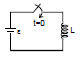circuit wants to rise upto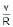in no time but inductor

opposes it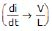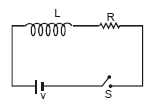hence at time t = 0 inductor will behave as an open circuit

at t = 0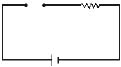As the time passes, i in the circuit rises and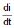decreases. At any instant t.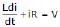current reaches the valueat time t = ∞ or we can say, inductor will behave as a simple wire.

at t = ∞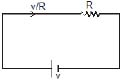Ex. 43 Find value of current i, i1 and i2 in given figure at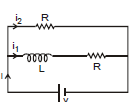(a) time t = 0

(b) time t = ∞

Ans: (a) At time t = 0 inductor behaves as open circuit

i = v/R

i1 = 0

i2= i = v/R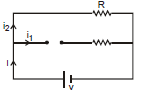(b) At time t = ∞. Inductor will behaves as simple wire

i =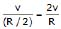i1 = i2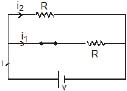9. GROWTH AND DECAY OF CURRENT IN L-R CIRCUIT:

9.1 Growth of Current:

Consider a circuit containing a resistance R, an inductance L, a two way key and a battery of e.m.f E connected in series as shown in figure. When the switch S is connected to a, the current in the circuit grows from zero value. The inductor opposes the growth of the current. This is due to the fact that when the current grows through inductor, a back e.m.f. is developed which opposes the growth of current in the circuit. So the rate of growth of current is reduced. During the growth of current in the circuit, let i be the current in the circuit at any instant t. Using Kirchhoff's voltage law in the circuit, we obtain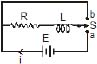E - L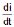= R i or E - Ri = Lor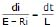Multiplying by - R on both the sides, we get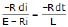Integrating the above equation, we have

loge(E - Ri) = -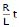+ A ...(1)

where A is integration constant. The value of this constant can be obtained by applying the condition that current i is zero just at start i.e., at t = 0.

Hence,

loge E = 0 + A

or A = logeE ...(2)

Substituting the value of A from equation (2) in equation (1), we get

loge(E - Ri) = -+ loge E

or loge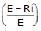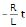or= exp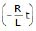or 1 -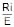= expor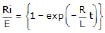Therefore,     i =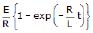The maximum current in the circuit i0 = E/R.

So,    i = i0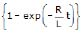...(3)

Equation (3) gives the current in the circuit at any instant t. It is obvious from equation (3) that i = i0, when

exp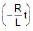= 0 i.e., at t = ∞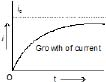Hence the current never attains the value i0 but it approaches it asymptotically. A graph between current and time is shown in figure.

We observe the following points

(i) When t = (L/R) then

i = i0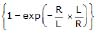= i0 {1 - exp(-1)} = i0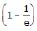= 0.63 i0

Thus after an interval of (L/R) second, the current reaches to a value which is 63% of the maximum current. The value of (L/R) is known as time constant of the circuit and is represented by t. Thus the time constant of a circuit may be defined as the time in which the current rises from zero to 63% of its final value. In terms of t,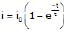(ii) The rate of growth of current (di/dt) is given by=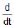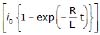⇒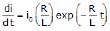...(4)

From equation (3), exp=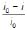Therefore,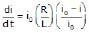=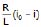...(5)

This shows that the rate of growth of the current decreases as i tends to i0. For any other value of current, it depends upon the value of R/L. Thus greater is the value of time constant, smaller will be the rate of growth of current.

Note: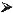Final current in the circuit =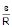, which is independent of L.After one time constant, current in the circuit=63% of the final current (verify yourself)More time constant in the circuit implies slower rate of change of current.If there is any change in the circuit containing inductor then there is no instantaneous effect on the flux of inductor.

L1i1 = L2i2

Ex. 44 At t = 0 switch is closed (shown in figure) after a long time suddenly the inductance of the inductor is made h times lesser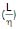than its initial value, find out instant current just after the operation.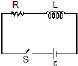Ans: Using above result (note 4)

L1i1 = L2i2 ⇒ i2 =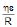Ex. 45 Which of the two curves shown has less time constant.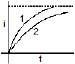Ans: curve 1

9.2 Decay of Current:

Let the circuit be disconnected from battery and switch S is thrown to point b in the figure. The current now begins to fall. In the absence of inductance, the current would have fallen from maximum i0 to zero almost instantaneously. But due to the presence of inductance, which opposes the decay of current, the rate of decay of current is reduced.

suppose during the decay of current, i be the value of current at any instant t. Using Kirchhoff's voltage law in the circuit, we get

- L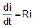or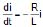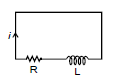Integrating this expression, we get

loge i = -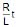+ B

where B is constant of integration. The value of B can be obtained by applying the condition that when t = 0, i = i0

Therefore, loge i0 = B

Substituting the value of B, we get

logei = -+ logei0

or loge= -or (i/i0) = exp...(6)

or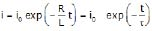where t = L/R = inductive time constant of the circuit.

It is obvious from equation that the current in the circuit decays exponentially as shown in figure.

We observe the following points

(i) After t = L/R, the current in the circuit is given by

i = i0 exp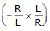= i0 exp(-1)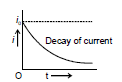= (i0 / e) = i0/2.718 = 0.37 i0

So after a time (L/R) second, the current reduces to 37% of the maximum current i0. (L/R) is known as time constant t. This is defined as the time during which the current decays to 37% of the maximum current during decay.

(ii) The rate of decay of current in given by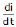=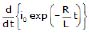⇒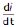=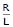i0 exp=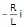...(7)

or -=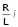This equation shows that when L is small, the rate of decay of current will be large i.e., the current will decay out more rapidly.

Ex. 46 In the following circuit the switch is closed at t = 0. Initially there is no current in inductor. Find out current the inductor coil as a function of time.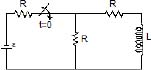Ans: At any time t

- e + i1 R - (i - i1) R = 0

- e + 2i1 R - i R = 0

i1 =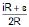Now, - e + iR + iR +L.= 0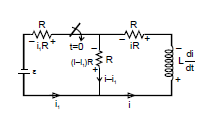- e +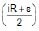+ iR + i.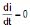⇒ -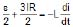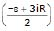dt = - L. di ⇒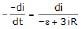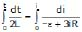⇒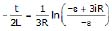- ln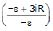=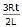i = +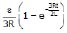Ex. 47 Figure shows a circuit consisting of a ideal cell, an inductor L and a resistor R, connected in series. Let the switch S be closed at t = 0. Suppose at t = 0 current in the inductor is i0 then find out equation of current as a function of time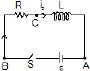Ans: Let an instant t current in the circuit is i which is increasing at the rate di/dt.

Writing KVL along the circuit, we have

ε - L- iR = 0 ⇒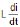= ε - iR

⇒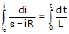⇒ ln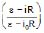= -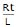⇒ ε - iR = (ε - i0R)e-Rt/L ⇒ i =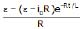Offer running on EduRev: Apply code STAYHOME200 to get INR 200 off on our premium plan EduRev Infinity!

## Physics For JEE

188 videos|346 docs|307 tests

,

,

,

,

,

,

,

,

,

,

,

,

,

,

,

,

,

,

,

,

,

;